# Tensor product spline surfaces

All spline curves studied before work well in space of any dimension. One need just take its control points in corresponding space. We used 2D plane as since it is enough and the simplest for computer representation. But spline surfaces are used mainly in 3D space (and IMHO Internet is too "plain" yet :) therefore I made several 3D spline models.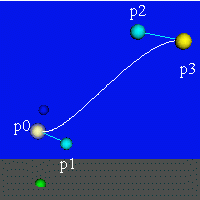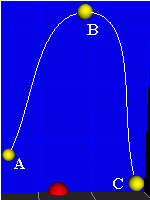Look at interactive cubic Bezier and Cardinal spline curves VRML demos. Controls. Press a control point (a ball) to mark it by the white semi-transparent sphere. Then drag mouse in the XY, YZ or XZ plane to move the point. The red ball in the Cardinal demo changes α (you see the α value in the "Status bar").

# Bezier surface

Bezier spline surface (or patch) S(u,v) is constructed as the tensor product of two Bezier curves
S(u,v) = ∑i=0,nj=0,m Bin(u) Bjm(v)Pij ,     0 ≤ u, v ≤ 1 .
where (n+1)(n+1) control points Pij make the control polyhedron of the surface (see the right picture below or VRML patch all non-interactive VRMLs use "native" NURBS and work under "Cortona" and "Contact"). You can think that the tensor product patch is made by moving the control points of one Bezier curve (the red curve in the left picture below) along a set of Bezier curves (the blue ones) to sweep out a surface, e.g.
S(u,v) = ∑i=0,n Bin(u) ∑j=0,m Bjm(v)Pij = ∑i=0,n Bin(u) Pi(v),     Pi(v) = ∑j=0,m Bjm(v)Pij .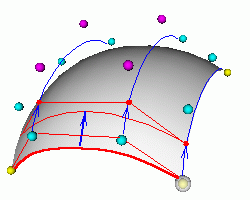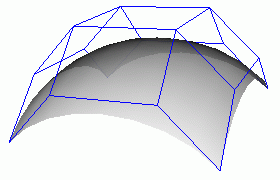Look at interactive bicubic Bezier surface VRML demo (2.5kb).
Controls. Move the control points as in the Curves demos. Press the red sphere to turn off/on IFS surface, the white sphere turns off/on ILS grid and the blue sphere prints 16 control points to the VRML Console panel.
Holger Grahn (Blaxxun Interactive) made the SurfNURBS demo with "native" NURBS for "Contact" and "Cortona" (press the orange sphere to turn off/on the Nurbs surface).

Noninteractive "native" 2x2 order (linear) Bezier patch. Isn't it amazing, that this "nonlinear" quadrilateral (its four points don't belong to a plane) is made of a line moved along two other lines? You see these lines in the wireframe image.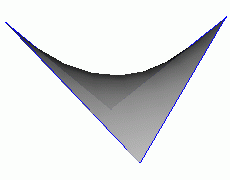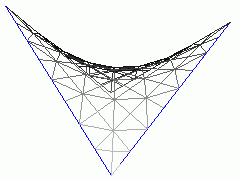In every point of a Bezier patch we have two tangents directions A = ∂S(u,v)/∂u and B = ∂S(u,v)/∂v. Therefore we get the normal directions N = [A B] to the surface, i.e.
Nx = (∂Sy /∂u)(∂Sz /∂v) - (∂Sz /∂u)(∂Sy /∂v),
Ny = (∂Sz /∂u)(∂Sx /∂v) - (∂Sx /∂u)(∂Sz /∂v),
Nz = (∂Sx /∂u)(∂Sy /∂v) - (∂Sy /∂u)(∂Sx /∂v).

We can normalize this vector next.

# NURBS surface

In a similar way NURBS surface S(u,v) is constructed as the tensor product of two NURBS curves
S(u,v) = ∑i=0,nuj=0,nv NiKu(u) NjKv(v)wijPij / ∑i=0,nuj=0,nv NiKu(u) NjKv(v)wij .
Look at quadratic patch with the control polyhedron. A NURBS patch with large nu, nv values can be very complex. A big texture can be mapped and fitted accurately to it. But a single patch is not enough to make a surface with complex topology (e.g. with holes or branches), therefore it is useful to study complex objects made of many patches further.

# SplineGlobe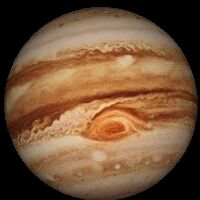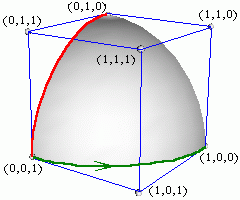It is evident, that many useful surfaces can be obtained as a single NURBS patch by tensor product of circular arcs and lines: cone, cylinder, hyperboloid, torus, ring and sphere.
Fig. illustrates how to make 3x3 points sphere segment of biquadratic NURBS. Its 3D and 4D homogeneous control points Pij are
 (0,1,0) (0,1,0) (0,1,0) (0,1,1) (1,1,1) (1,1,0) (0,0,1) (1,0,1) (1,0,0)
 (0,1,0, 1) (0,c,0, c) (0,1,0, 1) (0,c,c, c) (1/2,1/2,1/2, 1/2) (c,c,0, c) (0,0,1, 1) (c,0,c, c) (1,0,0, 1)
where c = cos 45 o = 2 -1/2 = 0.707.

If you map a mercator projection photo from NASA (Maps of the Solar System) on this sphere, you'll get "SplineGlobe".
I like Jupiter (it takes 115kb Jpeg from USA, unfortunately IE says nothing about downloading).

# Cardinal spline patches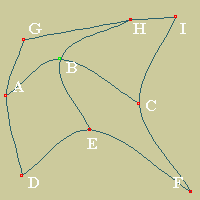Cardinal spline curve can be generalized easy for 2D regular net of quadrilateral patches. This generalization is evident for ABC and EBH splines. Therefore we get the four "boundary" control points around the B point (B+-ΔU) , (B+-ΔV) , where   ΔU = (C - A)/6 , ΔV = (H - E)/6. The next four "inner" control points are (B+-ΔU+-ΔV). It's a hint but not an explanation yet.

Contents   Previous: NURBS   Next: Points, Nets, Patches...
updated 24 August 2001     VRMLs tested under "Cortona 3.1" and "CosmoPlayer"